# AP Chemistry : VSEPR and Bond Hybridization

## Example Questions

### Example Question #81 : Bonding And Forces

What is the orbital hybridization of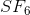?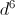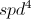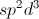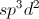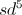Explanation:has six bonds and no lone pairs, so it has an octahedral geometry and its bonds are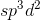hybridized.

### Example Question #31 : Vsepr And Bond Hybridization

What is the hybridization on the sulfur molecule in the following molecule?

H2S

s
sp3d
sp
sp2
sp3
Explanation:

the sulfur has 2 atoms bonded (the H) and 2 pairs of electrons, which is a total of 4 electron domains. The hybridization of the sulfur therefore is sp3

### Example Question #881 : Ap Chemistry

What is the hybridization of the molecular bonds and molecular geometry of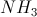?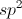hybridization and trigonal planar geometryhybridization and trigonal pyramidal geometry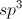hybridization and trigonal pyramidal geometryhybridization and trigonal planar geometryhybridization and trigonal pyramidal geometry

Explanation:

Nitrogen is a Group 5 element, so when it is bound to three hydrogen atoms, it will have a lone pair.has four areas of electron density (three bonds and one lone pair), so its bonds arehybridized.

Because of the lone pair,has a trigonal pyramidal geometry.

### Example Question #33 : Vsepr And Bond Hybridization

Which of the following molecules has an atom with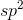hybridization?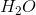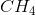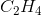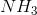Explanation:hybridization occurs when a double bond is present in a molecule. The only molecule that has a double bond is ethene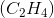. Both carbon atoms exhibithybridization.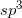hybridization is observed in atoms that have only single bonds, while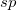hybridization occurs when a triple bond is present. The other given molecules all contain onlyhybridized atoms.

### Example Question #34 : Vsepr And Bond Hybridization

A carbonyl carbon is double bonded to an oxygen atom, as well as two other substituents. What type of hybrid orbitals are present on the carbonyl carbon?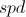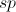Explanation:

Carbon hybrid orbitals are combinations of s and p valence orbitals, and are equal in energy. The hybridization of an atom is dependent on the number of atoms that it is bonded to, as well as the number of lone pairs on the atom in question. A carbonyl carbon is bonded to three other atoms (keep in mind the double bond is not counted twice!) and it has no lone electron pairs. As a result, a carbonyl carbon will exhibit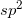hybridization.

A carbon with four substituents will be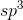hybridized. A carbon with a double bond will behybridized. A carbon with a triple bond or two double bonds will behybridized.

### Example Question #35 : Vsepr And Bond Hybridization

Molecule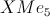has a trigonal bipyramidal shape. What type of hybridization describes its bonds?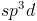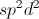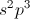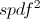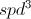Explanation:

Trigonal bipyramidal geometries arise from the hybridization of one s orbital, three p orbitals, and one d orbital, hence the name.

### Example Question #31 : Vsepr And Bond Hybridization

What is the maximum number of electrons an s subshell holds?Explanation:

Each s subshell has 2 electrons in it, each with opposing spin.

### Example Question #37 : Vsepr And Bond Hybridization

How many electrons are there in the p subshell?Explanation:

Each p subshell can have a maximum of 6 electrons in it. Note that there are three p orbitals, denoted px, py, and pzThese orbitals represent the plane in space in which they reside.

### Example Question #38 : Vsepr And Bond Hybridization

What is the maximum number of electrons in a d subshell?Explanation:

Remember that the d block involves the transition metals. There are 10 transition metal elements per row/period, and those 10 transition elements represent the maximum 10 possible electrons that can be held per d subshell. The d subshell has five orbitals, each with the potential to hold two electrons.

### Example Question #31 : Vsepr And Bond Hybridization

What is the maximum number of electrons in the f subshell?